Question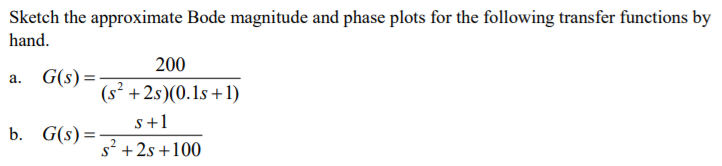We need at least 10 more requests to produce the answer.

0 / 10 have requested this problem solution

The more requests, the faster the answer.

All students who have requested the answer will be notified once they are available.

#### Earn Coins

Coins can be redeemed for fabulous gifts.

Similar Homework Help Questions
• ### For the following transfer function, sketch approximate straight-line Bode plots, including magnitude and phase plots. Show...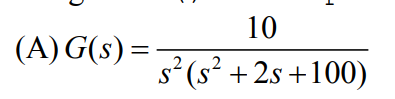For the following transfer function, sketch approximate straight-line Bode plots, including magnitude and phase plots. Show all steps clearly 10 4 (A)G(s)-7 s (s 2s +100)

• ### For the following closed-loop transfer functions, sketch the bode plots (magnitude and phase), id...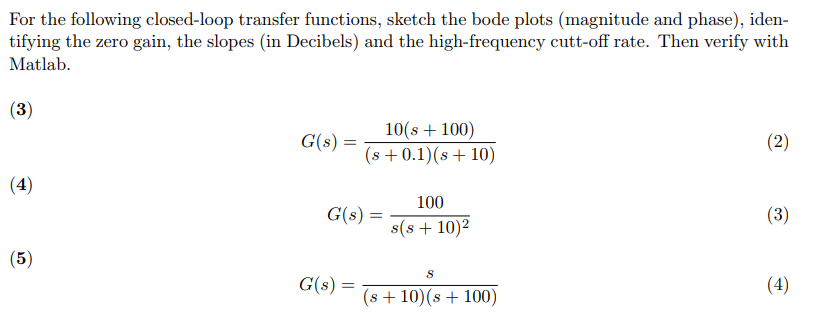For the following closed-loop transfer functions, sketch the bode plots (magnitude and phase), iden- tifying the zero gain, the slopes (in Decibels) and the high-frequency cutt-off rate. Then verify with Matlab C()101 100) s 0.1) (s 10) 100 s(s +10)2 G(s) = (56) G(s) = s+10(s+100) For the following closed-loop transfer functions, sketch the bode plots (magnitude and phase), iden- tifying the zero gain, the slopes (in Decibels) and the high-frequency cutt-off rate. Then verify with Matlab C()101 100) s...

• ### Problem 5: For the following transfer functions, sketch the bode asymptotic magnitude and phase p...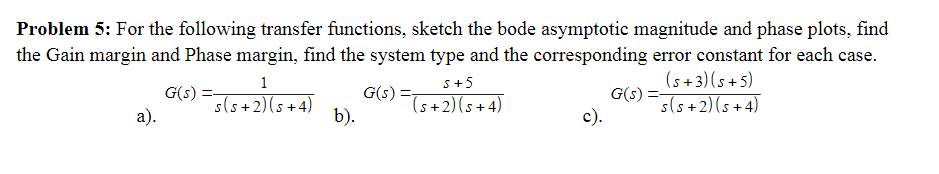Problem 5: For the following transfer functions, sketch the bode asymptotic magnitude and phase plots, find the Gain margin and Phase margin, find the system type and the corresponding error constant for each case. G(A) (s +3)(s +5) s(s +2) (s+4) S+5 2)b). Problem 5: For the following transfer functions, sketch the bode asymptotic magnitude and phase plots, find the Gain margin and Phase margin, find the system type and the corresponding error constant for each case. G(A) (s +3)(s...

• ### Bode Plots Sketch the Bode plot magnitude and phase for each of the three open-loop transfer...

Bode Plots Sketch the Bode plot magnitude and phase for each of the three open-loop transfer functions listed below. Verify your results using the bode m function in MATLAB.(a) $$G(s)=\frac{100}{s(0.1 s+1)(0.01 s+1)}$$(b) $$G(s)=\frac{1}{(s+1)^{2}\left(s^{2}+s+9\right)}$$(c) $$G(s)=\frac{16000 s}{(s+1)(s+100)\left(s^{2}+5 s+1600\right)}$$

• ### For the following closed-loop transfer functions, sketch the bode plots (magnitude and phase), id...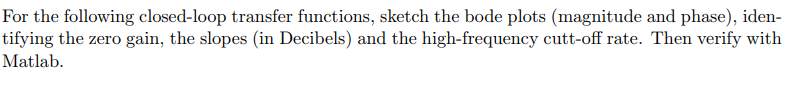For the following closed-loop transfer functions, sketch the bode plots (magnitude and phase), iden- tifying the zero gain, the slopes (in Decibels) and the high-frequency cutt-off rate. Then verify with Matlab (6) wn = 1, 〈 0.0.1, and 0.707. (8) Assuming the system of Problem 6 above, and an input of r(t) = 30sin(1000 t), use your bode plot to obtain the steady-state response For the following closed-loop transfer functions, sketch the bode plots (magnitude and phase), iden- tifying the...

• ### i) Draw the Bode plots (hand sketch, magnitude and phase!) for the following transfer function. Plot...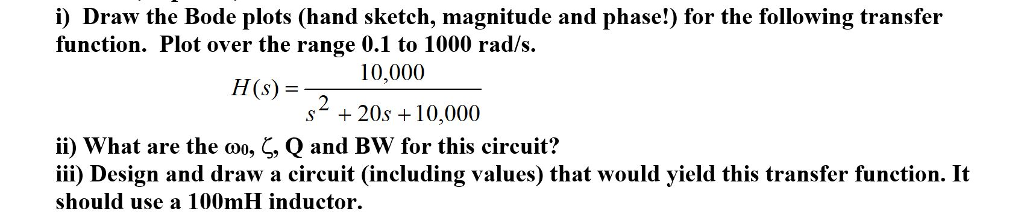i) Draw the Bode plots (hand sketch, magnitude and phase!) for the following transfer function. Plot over the range 0.1 to 1000 rad/s HS 10,000 (s) = s* + 20s 10,000 ii) what are the Q and Bw for this circuit? iii) Design and draw a circuit (including values) that would yield this transfer function. It should use a 100mH inductor , , Qano

• ### Sketch the Bode magnitude and phase plots for the following transfer function: G(s)=- a fimction: G(9)=...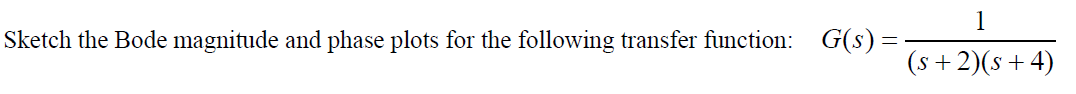Sketch the Bode magnitude and phase plots for the following transfer function: G(s)=- a fimction: G(9)= (s+2016+4) (s + 2)(+4)

• ### 0.1s(s 50)(s 200) G(s) (s+2)(s 2s+100) Consider the transfer function above a) Draw the Bode diagrams...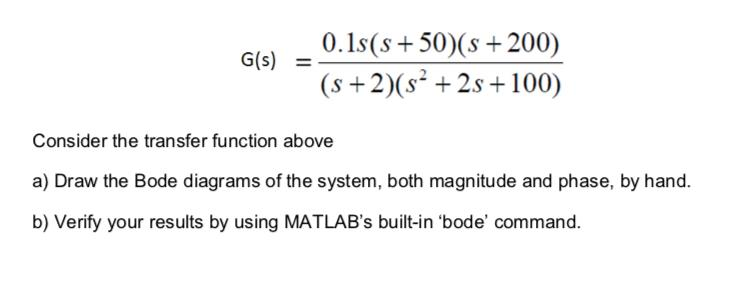0.1s(s 50)(s 200) G(s) (s+2)(s 2s+100) Consider the transfer function above a) Draw the Bode diagrams of the system, both magnitude and phase, by hand. b) Verify your results by using MATLAB's built-in 'bode' command. 0.1s(s 50)(s 200) G(s) (s+2)(s 2s+100) Consider the transfer function above a) Draw the Bode diagrams of the system, both magnitude and phase, by hand. b) Verify your results by using MATLAB's built-in 'bode' command.

• ### 2) Hand sketch the asymptotes of the Bode plot magnitude and phase for the following open-loop...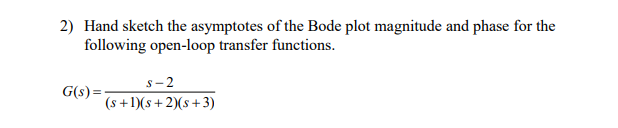2) Hand sketch the asymptotes of the Bode plot magnitude and phase for the following open-loop transfer functions. G()=— -2 (3) (s + 1)(+2)(8+3)

• ### 1- For each transfer function below, sketch the Bode magnitude and phase plots, a) T(s) 3040S...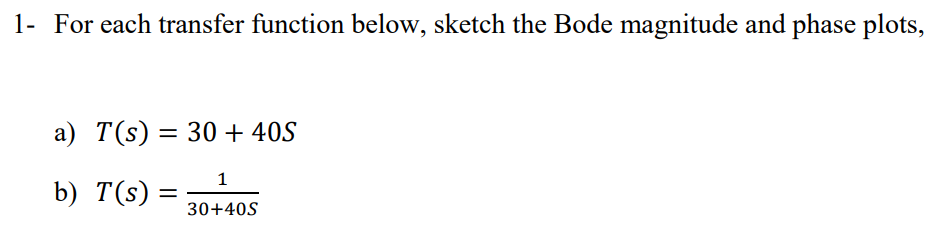1- For each transfer function below, sketch the Bode magnitude and phase plots, a) T(s) 3040S b) T(S) 30-405